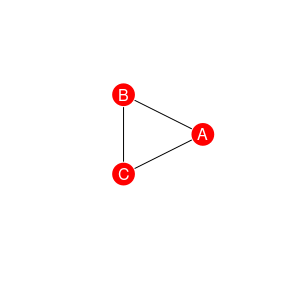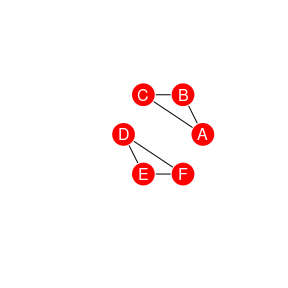# Getting Started with rnetcarto

#### 2023-01-17

## Loading required package: igraph
##
## Attaching package: 'igraph'
## The following objects are masked from 'package:stats':
##
##     decompose, spectrum
## The following object is masked from 'package:base':
##
##     union

# Rnetcarto in 60 seconds.

Rnetcarto provides fast network modularity and roles computation by simulated annealing (rgraph C library wrapper for R).

It exposes one main command named netcarto that take a graph as an input (formatted as an adjacency matrix or list, as described in more detail below) and returns a partition of the graph optimizing a given modularity criterion. It also computes the modularity roles of the nodes.

Here is a small example:

 # Generate a simple random network
a = matrix(as.integer(runif(100)<.3), ncol=10)
a[lower.tri(a)] = 0
rownames(a) = c('a','b','b','c','d','e','f','g','h','i')
colnames(a) = rownames(a)
# Find an optimal partition for modularity using netcarto.
#  The output consists in a table containing node properties,
#  and the modularity value of the partition.
netcarto(a)
## []
##    name module connectivity participation             role
## 4     c      0   -1.0000000     0.0000000 Ultra peripheral
## 5     d      0    1.0000000     0.6250000        Connector
## 2     b      1   -1.2247449     0.0000000 Ultra peripheral
## 8     g      1   -1.2247449     0.0000000 Ultra peripheral
## 1     a      1    0.8164966     0.3200000       Peripheral
## 6     e      1    0.8164966     0.3200000       Peripheral
## 10    i      1    0.8164966     0.3200000       Peripheral
## 7     f      2   -0.7071068     0.0000000 Ultra peripheral
## 3     b      2   -0.7071068     0.6666667        Connector
## 9     h      2    1.4142136     0.4444444       Peripheral
##
## []
##  0.1373457

# Input: How should I format my data?

The netcarto function can read network in either adjacency matrix or adjacency list format.

## Matrix format

square symmetric matrix. In this format, the weight $$w$$ of an between If you choose the matrix format, your network must consist in a vertices $$i$$ and $$j$$ is given by the corresponding value in the matrix web[i,j]. Auto-loop (i.e. diagonal terms are authorised). You may name the rows and/or columns, those names will be used in the function output. Example:

### Example 1: Triplet

    input = matrix(0,3,3)
input[1,2] = 1
input[2,3] = 1
input[3,1] = 1
input[2,1] = 1
input[3,2] = 1
input[1,3] = 1
rownames(input) = c("A","B","C")
colnames(input) = rownames(input)
print(input)
##   A B C
## A 0 1 1
## B 1 0 1
## C 1 1 0

Note that igraph package can be used to manipulate and plot graphs:

    # import from rnetcarto matrix format to igraph:
# Export to a matrix compatible with netcarto:
input = igraph::get.adjacency(G,sparse=FALSE)### Example 2: Two triplets

    input = matrix(0,7,7)
input[1,2] = 10
input[2,3] = 10
input[3,1] = 10
input[4,5] = 10
input[5,6] = 10
input[6,4] = 10
rownames(input) = c("A","B","C","D","E","F","G")
colnames(input) = rownames(input)

Note that:

• Empty columns and lines are removed (Here G).
• If the matrix is not symmetric, symmetry will be enforced by taking web = web+t(web)-diag(web)

So the previous matrix is equivalent to:

##    A  B  C  D  E  F
## A  0 10 10  0  0  0
## B 10  0 10  0  0  0
## C 10 10  0  0  0  0
## D  0  0  0  0 10 10
## E  0  0  0 10  0 10
## F  0  0  0 10 10  0### Example 3: Bipartite triplets

Note that the matrix may not be square and symmetric if and only if you are considering a bipartite network (using the bipartite flag).

    input = matrix(0,6,2)
input[1,1] = 1
input[2,1] = 1
input[3,1] = 1
input[4,2] = 1
input[5,2] = 1
input[6,2] = 1
rownames(input) = c("A","B","C","D","E","F")
colnames(input) = c("Team 1", "Team 2")
print(input)
##   Team 1 Team 2
## A      1      0
## B      1      0
## C      1      0
## D      0      1
## E      0      1
## F      0      1

## List format

If you choose the list format, your network must be formatted as a R-list. The first element must be a vector giving the label. The third element is a vector of the edge weights. The weights are optional and are all set to one if the list contains only the first two elements.

### Example 1: Unweighted network:

    nd1 = c("A","B","C","D","E","F","C")
nd2 = c("B","C","A","E","F","D","D")
web = list(nd1,nd2,weights)
print(list(nd1,nd2))
## []
##  "A" "B" "C" "D" "E" "F" "C"
##
## []
##  "B" "C" "A" "E" "F" "D" "D"

### Example 2: Weighted network

    nd1 = c("A","B","C","D","E","F","C","A")
nd2 = c("B","C","A","E","F","D","D","D")
weights = c(10,10,10,10,10,10,10,10,1)
web = list(nd1,nd2,weights)
print(web)
## []
##  "A" "B" "C" "D" "E" "F" "C" "A"
##
## []
##  "B" "C" "A" "E" "F" "D" "D" "D"
##
## []
##  10 10 10 10 10 10 10 10  1

### Example 3: Bipartite network

    nd1 = c("A","B","C","D","E","F","C","A")
nd2 = c("Team1","Team2","Team1","Team1","Team2","Team1","Team1","Team2")
bipartite = list(nd1,nd2)
print(bipartite)
## []
##  "A" "B" "C" "D" "E" "F" "C" "A"
##
## []
##  "Team1" "Team2" "Team1" "Team1" "Team2" "Team1" "Team1" "Team2"

# Output: How should I read the result?

The netcarto command output a list. Its first element is a dataframe giving the name module, connectivity, and participation coefficient for each node of the input graph. The second element is the modularity of this optimal partition.

### Example 1: Weighted network

    netcarto(igraph::get.adjacency(G,sparse=FALSE))
## []
##   name module connectivity participation             role
## 4    D      0            0             0 Ultra peripheral
## 5    E      0            0             0 Ultra peripheral
## 6    F      0            0             0 Ultra peripheral
## 1    A      1            0             0 Ultra peripheral
## 2    B      1            0             0 Ultra peripheral
## 3    C      1            0             0 Ultra peripheral
##
## []
##  0.5

### Example 2: Bipartite network

   netcarto(bipartite, bipartite=TRUE)
## []
##   name module connectivity participation             role
## 4    D      0   -0.5773503     0.0000000 Ultra peripheral
## 6    F      0   -0.5773503     0.0000000 Ultra peripheral
## 1    A      0   -0.5773503     0.4444444       Peripheral
## 3    C      0    1.7320508     0.0000000 Ultra peripheral
## 2    B      1    0.0000000     0.5000000       Peripheral
## 5    E      1    0.0000000     0.5000000       Peripheral
##
## []
##  0.3317308